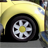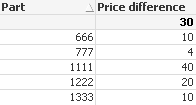# QlikView App Dev

Discussion Board for collaboration related to QlikView App Development.

Announcements
Support Cases coming to Qlik Community Oct. 4! Start chats, open cases, explore resources: READ DETAILS
cancel
Showing results for
Did you mean:Contributor

## Need Help for an Intersection Problem in Set Analysis

Hi at all,

i have a problem with an intersection in Set Analysis.

I have one Table with Parts, Prices and Factorys.

I need the difference beetween the Prices in two different Factories for the Same Part.

How is the correct Expression with Aggr in a Text Field?

Details in the Example (.qvw)

10 RepliesMVP

=IF(sum({<Factory= {'200'}>} Price) > sum({<Factory= {'100'}>} Price), sum({<Factory= {'200'}>} Price)- sum({<Factory= {'100'}>} Price))

or

=Aggr(IF(sum({<Factory= {'200'}>} Price) > sum({<Factory= {'100'}>} Price), sum({<Factory= {'200'}>} Price)- sum({<Factory= {'100'}>} Price)),Part, Factory)MVP

=If( (sum({<Factory= {'200'}>} Price) -sum({<Factory= {'100'}>} Price))>0,sum({<Factory= {'200'}>} Price) -sum({<Factory= {'100'}>} Price))

PFASpecialist III

Dear ,

can you try like

=if(sum({<Factory= {'200'}>} Price)>sum({<Factory= {'100'}>} Price),(sum({<Factory= {'200'}>} Price))-(sum({<Factory= {'100'}>} Price)))Contributor
Author

yes thank you. but i need an aggr expression in a textbox for the resultonly if PART exist in Factory 100 and PART exist in Factory 200 then make the Price Difference #

the result is the SUM: in this exampe 10+4 = 14MVP

=SUM(Aggr(IF(sum({<Factory= {'200'}>} Price) > sum({<Factory= {'100'}>} Price), sum({<Factory= {'200'}>} Price)- sum({<Factory= {'100'}>} Price)),Part, Factory))Contributor
Author

thank you, but the result of your expression is "175" ... the correct result is 14 (screenshot)MVP

Can you elaborate little more what are expecting ?

ThanksContributor
Author

I need the price difference beetween the parts who exist in Factory 100 and Factory 200.

Example:

the part 666 and 777 exist in both Factorys but in factory 200 is the part 666 and 777 more expensive.

i need an expression for following conditions:

- Parts are the SAME - Part exist in both factorys

- If in Factory 200 the price is higher than in Factory 100 then i need the sum

for this example is 14 (see screenshot above)MVP

Use this...

=IF(SUM({<Factory = {'200'}, Part = P({1<Factory = {'100'}>})>}Price) > SUM({<Factory = {'100'}, Part = P({1<Factory = {'200'}>})>}Price),

SUM({<Factory = {'200'}, Part = P({1<Factory = {'100'}>})>}Price) - SUM({<Factory = {'100'}, Part = P({1<Factory = {'200'}>})>}Price))Community Browser